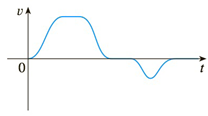Chapter 3.9, Problem 48E

Chapter
Section
Textbook Problem

# The graph of the velocity function of a particle is shown in the figure. Sketch the graph of a position function.To determine

To draw:

The graph of a position function from the graph of the velocity function.

Explanation

Explanations:

1) Concept:

A position function is an antiderivative of a velocity function. Therefore, to plot the graph of the position function, plot the graph of antiderivative of the given graph.

2) Calculation:

The position function is an antiderivative to velocity.

Let F(x) be a position function, so when velocity is positive, Fx  is increasing.

When the velocity is increasing, function F F(x) is concave upward

### Still sussing out bartleby?

Check out a sample textbook solution.

See a sample solution

#### The Solution to Your Study Problems

Bartleby provides explanations to thousands of textbook problems written by our experts, many with advanced degrees!

Get Started